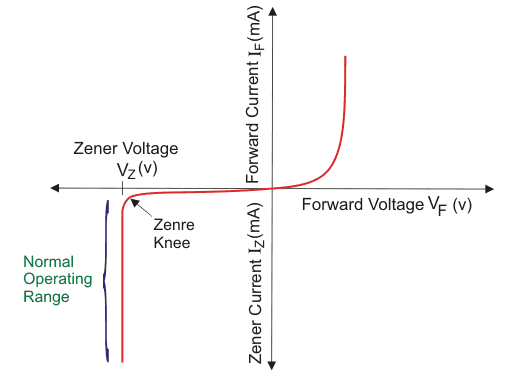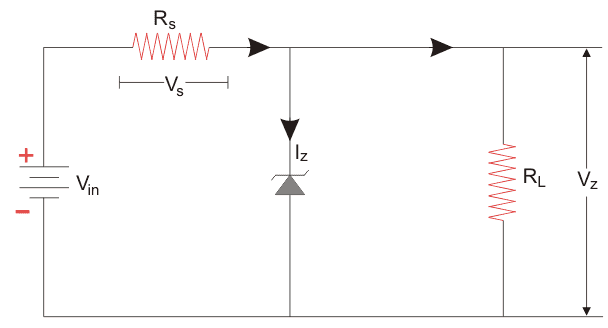# Zener Diode as Voltage Regulator

A Zener diode is one of the specially designed diodes that predominately works in reverse biased conditions. They are more heavily doped than ordinary diodes, due to which they have narrow depletion region. While regular diodes get damaged when the voltage across them exceeds the reverse breakdown voltage, Zener diodes work exclusively in this region. The depletion region in Zener diode goes back to its normal state when the reverse voltage gets removed. This particular property of Zener diodes makes it useful as a voltage regulator. Let us see how this happens.

Let us first comprehend how a Zener diode works before we understand Zener diode as a voltage regulator.When we apply a reverse voltage to a Zener diode, a negligible amount of current flows through the circuit. When a voltage higher than Zener breakdown voltage is applied, Zener breakdown occurs. Zener breakdown is a phenomenon where a significant amount of current flows through the diode with a negligible drop in voltage. When we increase the reverse voltage further, the voltage across the diode remains at the same value of Zener breakdown voltage whereas the current through it keeps on rising as seen in the graph above. Here in the graph Vz refers to the Zener breakdown voltage. Zener breakdown voltage typically can range from 1.2 V to 200 V depending on its application.

The exciting part of this diode is that we can choose the Zener diode with a suitable breakdown voltage to work as a voltage regulator in our circuit. For example, we want that the voltage across a load in our circuit does not exceed, let’s say, 12 volts. Then we can select a Zener diode with a breakdown voltage of 12 volts and connect it across the load. Then even if the input voltage exceeds that value, the voltage across the load will never exceed 12 volts.
Let us try to understand that with a circuit diagramHere the Zener diode is connected across the load RL. We want the voltage across the load to be regulated and not cross the value of Vz. Depending on our requirement, we choose the suitable Zener diode with a Zener breakdown voltage near to the voltage we require across the load. We connect the Zener diode in reverse bias condition. When the voltage across the diode exceeds the Zener breakdown voltage, a significant amount of current starts flowing through the diode. As the load is in parallel to the diode, the voltage drop across the load is also equal to the Zener breakdown voltage. The Zener diode provides a path for the current to flow and hence the load gets protected from excessive currents. Thus the Zener diode serves two purposes here: Zener diode as a voltage regulator as well as it protects the load from excessive current.

Want To Learn Faster? 🎓
Get electrical articles delivered to your inbox every week.
No credit card required—it’s 100% free.